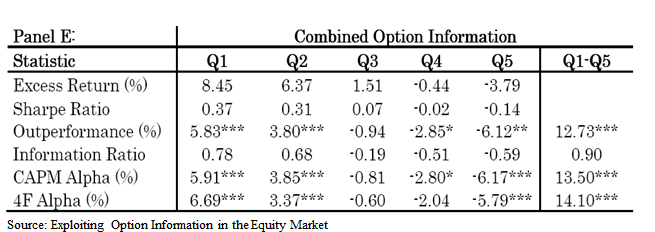### Exploiting Option Information in the Equity Market

Guido Baltussen, Bart Van Der Grient, Wilma De Groot, Erik Hennink and Weili Zhou

### Key Points:

• Long/Short Value-Weighted alpha estimates of 118bp monthly. Sample covers data from 1996 to 2009.
• Values come from information diffusions between stocks and options.

##### Abstract:

Public option market information contains exploitable information for equity investors for an investable universe of liquid large-cap stocks. Strategies based on several option measures predict returns and alphas on the underlying stock. Transaction costs are an important factor given the high turnover of these strategies, but significant net alphas can be obtained when using a simple transaction cost reducing approach. These findings suggest that information diffuses from the option market into the underlying stock market.

### Strategy Summary:

1. Calculate OTM skew, ATM skew, Changes in ATM skew and Spreads of realized and implied volatility.
2. Calculate z-scores for each variables. (Z-score of a variable is constructed by subtracting its cross-sectional median from the values of the variable and dividing by its median absolute deviation)
3. Sort 1,250 largest stocks into quintile portfolios base on average z-scores.
4. Construct a value-weighted portfolio by longing stocks in the first quintile and shorting stocks in the last quintile.
5. Do a weekly rebalance and make money.

### To juice up:

1. Calculate Z-scores for each variable then sort stocks base on average Z-scores.
2. Use decile portfolios instead of quintiles and earn monthly alpha of 1.1%;
3. Use value-weighted portfolios and quintiles to earn monthly alpha of 1.18%.